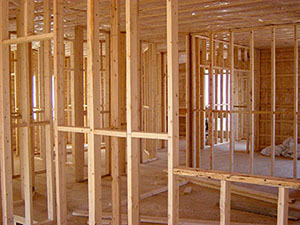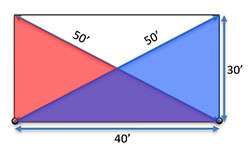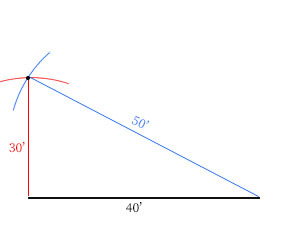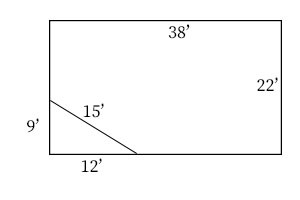• ### Browse All Lessons

##### Assign Lesson

Help Teaching subscribers can assign lessons to their students to review online!

 Tweet# Construction - Squaring a Foundation or WallWhen constructing parts of a building it is extremely important to have accurate angles. The most common angle is a $90deg$ corner. The process of squaring a foundation, wall, or any other component does not mean literally making it square but, making sure that it has square corners. In this lesson you will learn 3 different ways of doing this using very basic tools.

All methods use a right triangle as the key to ensuring $90deg$ angles.Trial and error can be very effective if you have relatively small measurements and have a few people to help. Let's imagine that you are building a wall. When you nail the studs and plates together you will have an approximate rectangle, but not necessarily have 4 right-angle corners. If you imagine 2 identical right triangles that share the base of the wall but each use opposite sides then the hypotenuses will form an X through the middle of the wall. If both triangles are congruent then they will have hypotenuses which are the same length. If the first 2 corners are anchored and tape measures hooked on those corners and run diagonally, then each person can call out their measurement while the top is being slid sideways until both tape measures are reading the same length. At this point the wall will be square.Using Pythagorean Theorem is often the most effecient method. As an example, let's say we are laying out a foundation 30' X 40'. Using $A^2 + B^2 = C^2$ we can determine that the diagonal of the foundation will be 50'. If you measure the side and mark an arc on the ground and then measure the diagonal and marc an arc for it as well then the corner will be where the two arcs intersect. This intersection of the arcs is where the side is 30' and the diagonal is 50' which we know will create a right triangle. This can be repeated for the other corner. This method is very accurate and easy to do with just one person.

The third method uses a compass and trigonometry. This method is not particularly easy but can be useful in some situations, especially when the corner pegs for a foundation will be at different elevations. Using trigonometric ratios you can find the angles for the diagonals and then add or subtract these from the magnetic bearing of the edges.Quick Tip:
The 3-4-5 triangle is very convenient when squaring objects. Any triangle whose sides are in the ratio 3-4-5 will be a right triangle. In the example above we used 30', 40', and 50'. It would also work as 6-8-10, 9-12-15, 12-16-20, or any other multiple. Another advantage of doing this is that you can square a very large foundation with a relatively small tape measure. Even if your finished construction is not in these dimensions you can still use it to square the corner. In the illustration, you can see a 9-12-15 triangle being used to square a foundation that is 22' X 38'. This method will not always be quite as precise as measuring the full diagonal.

Directions for This Lesson: